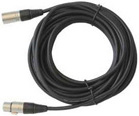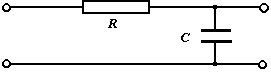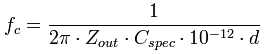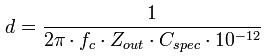Deutsche VersionCalculation of Cable Attenuation (Loss)
Cable Length and Cable Capacitance
Treble Loss (Attenuation) and Cutoff Frequency
Line capacitance and Line calculation
Cut-off frequency = corner frequency = crossover frequency
= half-power frequency = 3 dB frequency = break frequency is all the same.This is not about coaxial cables, antennas and satellite frequencies. Here we have a look at a normal audio, mic or microphone cable.

 Cable and treble attenuation    treble cut filter (low pass)    Simplified principle C = Cspec · dA frequently asked question is at which cutoff frequency fc the microphone cable has a (−) 3 dB loss of treble? Which treble frequencies are attenuated? Which length d a cable can have to reach this attenuation? How are high frequencies attenuated by the length of the cable? Cables are characterized by the capacity of the cable between the conductors, the resistance, inductance of the cable along the conductor, and the current crowding, which boost the resistance at high frequencies. The microphone cable usually has a capacitance from wire to wire of about Cspec = 100 pF per meter. The line resistance, and the inductance is usually negligible in practice. Each wire has an unavoidable cable capacitance, that leads to the attenuation of high frequencies, called cable loss. Because the input resistance (load) is large against the small output impedance (source), the input impedance (load) can be neglected.

Calculating the treble cutoff frequency fc of a cable

 Source impedance Zout ohms Capacitance C per m pF/m Cable length d meters ↓ Cutoff frequency fc Hz
 fc = Cutoff frequency at (−)3 dB treble loss in Hz Zout = Output impedance of microphone, source impedance Cspec = Specific capacitance of the cable in pF per m cable length d = Cable length of the cable in m            C = Cspec × d 1 pF (Picofarad) = 10−12 F (Farad)

 Formula for the cutoff frequency fc of the (−)3 dB treble attenuation:Another frequently asked question is of how long can the microphone cable be,
without having treble loss?

Cable length or line length

Calculation of the length of a cable at 3 dB treble attenuation

 Source impedance Zout ohms Capacitance C per m pF/m Cutoff frequency fc Hz ↓ Cable length d meters
 d = Cable length in m fc = Cutoff frequency at (−)3 dB treble loss in Hz Zout = Output impedance of microphone, source impedance Cspec = Capacitance in pF per m cable length     C = Cspec × d 1 pF (Picofarad) = 10−12 F (Farad)

 Formula for the length of the cable d and a given cutoff frequency fc:If you want to know how long the cable can be and permit a level attenuation of only 1 dB, you need to enter the double value of the cutoff frequency.

 Cable characteristic impedance is a cable characteristics which is only valid for radio frequency signals. Multimeters use DC current for resistance measurements, so you cannot measure the cable impedance using your multimeter or other simple measurement equipment.

Capacitance C, frequency f, and capacitive reactance XC

Converting feet to meters and vice versa
for U.S.A only. It's better to go metric - like the whole world.

 To use the calculator, simply enter a value. The calculator works in both directions of the ↔ sign.

 Feet (ft)  ft ↔ Meters (m)  m 1 foot = 0.3048 m                                 1 meter = 3.280839895 ft

Cable, wire, and wiring - Conversion of diameter to cross-sectional area